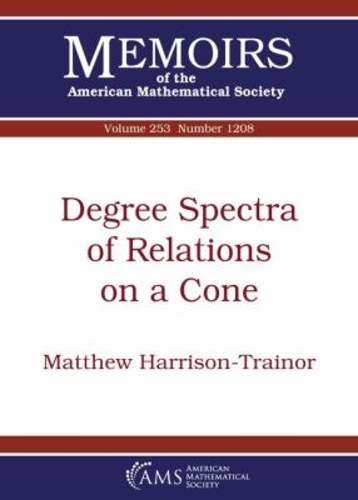•# Degree Spectra of Relations on a Cone - Memoirs of the American Mathematical Society (Paperback)

(author)
£74.50
Paperback 107 Pages / Published: 30/06/2018
• In stock
• Free UK delivery

In stock online

Your local Waterstones may have stock of this item. Please check by using Click & Collect
Let $\mathcal A$ be a mathematical structure with an additional relation $R$. The author is interested in the degree spectrum of $R$, either among computable copies of $\mathcal A$ when $(\mathcal A,R)$ is a natural'' structure, or (to make this rigorous) among copies of $(\mathcal A,R)$ computable in a large degree d. He introduces the partial order of degree spectra on a cone and begin the study of these objects. Using a result of Harizanov--that, assuming an effectiveness condition on $\mathcal A$ and $R$, if $R$ is not intrinsically computable, then its degree spectrum contains all c.e. degrees--the author shows that there is a minimal non-trivial degree spectrum on a cone, consisting of the c.e. degrees.

Publisher: American Mathematical Society
ISBN: 9781470428396
Number of pages: 107
Dimensions: 254 x 178 mm